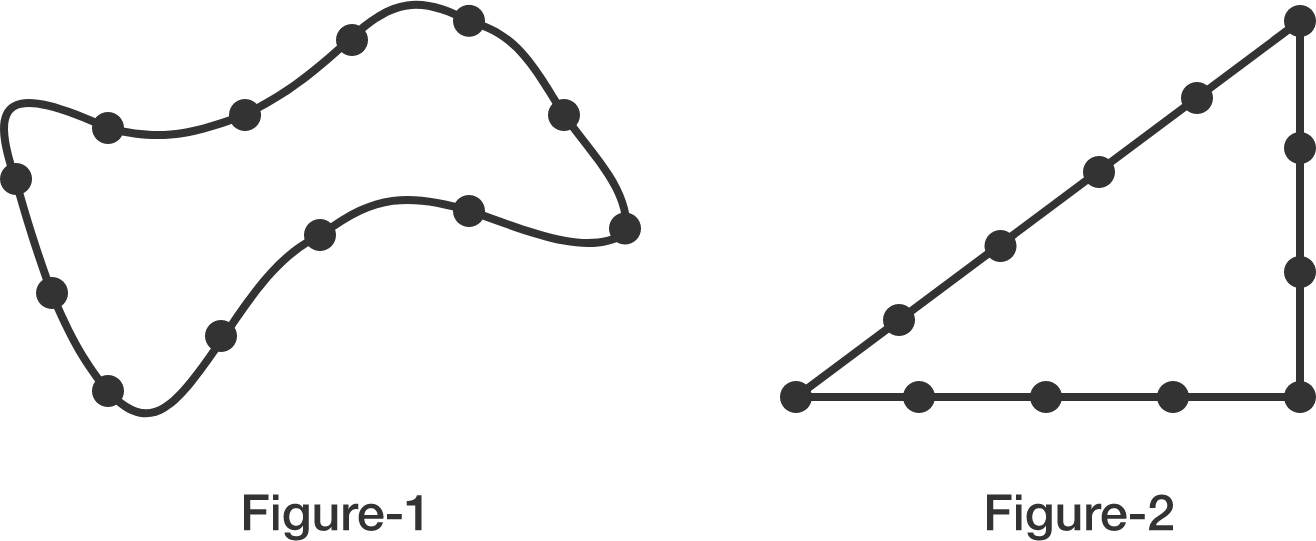# Knots and Pythagorean TrianglesThere are equally spaced $12$ knots in the loop of string in Figure-1. This string loop can be transformed into Figure-2, which is a right triangle with a knot on each of its vertices.

Does this same transformation work for any other number of knots $k\neq12$?

Find the minimum $k>12$.
If there is no such $k>12$, enter 0.

×

Problem Loading...

Note Loading...

Set Loading...